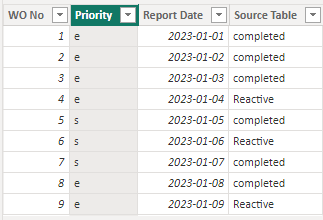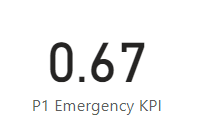cancel
Showing results for
Did you mean:Helper II

## Amend current DAX Measure to give previous month values

Hi Experts

how can i change the following measure to give me previous month

P1 Emergency KPI =
VAR ClosedP1Jobs = CALCULATE(COUNT('All Data'[WO No]), filter('All Data', and('All Data'[Priority]="e", month('All Data'[Report Date]) = month(now())) && 'All Data'[Source Table] = "completed"))

VAR p1JobsMTD = CALCULATE(count('All Data'[WO No]), filter('All Data', and('All Data'[Priority]="e", and(or('All Data'[Source Table] = "Reactive", 'All Data'[Source Table] = "Completed"), month('All Data'[Report Date]) = month(now() )))))

return closedp1Jobs/p1JobsMTd
1 ACCEPTED SOLUTIONCommunity Support

Hi  @apple1111 ,

I created some data:Here are the steps you can follow：

1. Create measure.

``````P1 Emergency KPI =
VAR ClosedP1Jobs =
CALCULATE (
COUNT ( 'All Data'[WO No] ),
FILTER (
ALL ( 'All Data' ),
AND (
'All Data'[Priority] = "e",
'All Data'[Report Date]
>= DATE ( YEAR ( TODAY () ), MONTH ( TODAY () ) - 1, 1 )
&& 'All Data'[Report Date]
<= EOMONTH ( DATE ( YEAR ( TODAY () ), MONTH ( TODAY () ) - 1, 1 ), 0 )
&& 'All Data'[Source Table] = "completed"
)
)
)
VAR p1JobsMTD =
CALCULATE (
COUNT ( 'All Data'[WO No] ),
FILTER (
'All Data',
OR (
'All Data'[Source Table] = "Reactive",
'All Data'[Source Table] = "Completed"
)
&& AND (
'All Data'[Report Date]
>= DATE ( YEAR ( TODAY () ), MONTH ( TODAY () ) - 1, 1 ),
'All Data'[Report Date]
<= EOMONTH ( DATE ( YEAR ( TODAY () ), MONTH ( TODAY () ) - 1, 1 ), 0 )
&& 'All Data'[Priority] = "e"
)
)
)
RETURN
DIVIDE ( ClosedP1Jobs, p1JobsMTD )
``````

2. Result:Best Regards,

Liu Yang

If this post helps, then please consider Accept it as the solution to help the other members find it more quickly

2 REPLIES 2Community Support

Hi  @apple1111 ,

I created some data:Here are the steps you can follow：

1. Create measure.

``````P1 Emergency KPI =
VAR ClosedP1Jobs =
CALCULATE (
COUNT ( 'All Data'[WO No] ),
FILTER (
ALL ( 'All Data' ),
AND (
'All Data'[Priority] = "e",
'All Data'[Report Date]
>= DATE ( YEAR ( TODAY () ), MONTH ( TODAY () ) - 1, 1 )
&& 'All Data'[Report Date]
<= EOMONTH ( DATE ( YEAR ( TODAY () ), MONTH ( TODAY () ) - 1, 1 ), 0 )
&& 'All Data'[Source Table] = "completed"
)
)
)
VAR p1JobsMTD =
CALCULATE (
COUNT ( 'All Data'[WO No] ),
FILTER (
'All Data',
OR (
'All Data'[Source Table] = "Reactive",
'All Data'[Source Table] = "Completed"
)
&& AND (
'All Data'[Report Date]
>= DATE ( YEAR ( TODAY () ), MONTH ( TODAY () ) - 1, 1 ),
'All Data'[Report Date]
<= EOMONTH ( DATE ( YEAR ( TODAY () ), MONTH ( TODAY () ) - 1, 1 ), 0 )
&& 'All Data'[Priority] = "e"
)
)
)
RETURN
DIVIDE ( ClosedP1Jobs, p1JobsMTD )
``````

2. Result:Best Regards,

Liu Yang

If this post helps, then please consider Accept it as the solution to help the other members find it more quicklySuper User

@apple1111 , if you need based on today

MTD Today =
var _min = eomonth(today(),-1)+1
var _max = today()
return
CALCULATE([Net], FILTER('Date','Date'[Date] >=_min && 'Date'[Date] <= _max))

LMTD Today=
var _min = eomonth(today(),-2)+1
var _max1 = today()
var _max = date(year(_max1),month(_max1)-1, day(_max1))
return
CALCULATE([Net], FILTER('Date','Date'[Date] >=_min && 'Date'[Date] <= _max))

LYMTD Today =
var _min = eomonth(today(),-13)+1
var _max1 = today()-1
var _max = date(year(_max1)-1,month(_max1), day(_max1))
return CALCULATE([Net], FILTER('Date','Date'[Date] >=_min && 'Date'[Date] <= _max))

MTD Yesterday =
var _max = today() -1
var _min = eomonth(_max,-1)+1
return CALCULATE([Net], FILTER('Date','Date'[Date] >=_min && 'Date'[Date] <= _max))

This Month Today =
var _min = eomonth(today(),-1)+1
var _max = eomonth(today(),0)
return CALCULATE([Net], FILTER('Date','Date'[Date] >=_min && 'Date'[Date] <= _max))

Last Month Today =
var _min = eomonth(today(),-2)+1
var _max = eomonth(today(),-1)
return CALCULATE([Net], FILTER('Date','Date'[Date] >=_min && 'Date'[Date] <= _max))

Same Month Last Year Today =
var _min = eomonth(today(),-13)+1
var _max = eomonth(today(),-12)
return CALCULATE([Net], FILTER('Date','Date'[Date] >=_min && 'Date'[Date] <= _max))

If you need based on selected date use time intelligence

MTD Sales = CALCULATE(SUM(Sales[Sales Amount]),DATESMTD('Date'[Date]))
last MTD Sales = CALCULATE(SUM(Sales[Sales Amount]),DATESMTD(dateadd('Date'[Date],-1,MONTH)))
last month Sales = CALCULATE(SUM(Sales[Sales Amount]),previousmonth('Date'[Date]))
MTD Sales = CALCULATE(SUM(Sales[Sales Amount]),DATESMTD('Date'[Date]))
last MTD Sales = CALCULATE(SUM(Sales[Sales Amount]),DATESMTD(dateadd('Date'[Date],-1,MONTH)))
last month Sales = CALCULATE(SUM(Sales[Sales Amount]),previousmonth('Date'[Date]))

Or try offset -

Power BI Offset Compare Categories, Time Intelligence MOM, QOQ, and YOY: https://youtu.be/5YMlkDNGr0U

Time Intelligence, Part of learn Power BI https://youtu.be/cN8AO3_vmlY?t=27510
Time Intelligence, DATESMTD, DATESQTD, DATESYTD, Week On Week, Week Till Date, Custom Period on Period,
Custom Period till date: https://youtu.be/aU2aKbnHuWs&t=145s